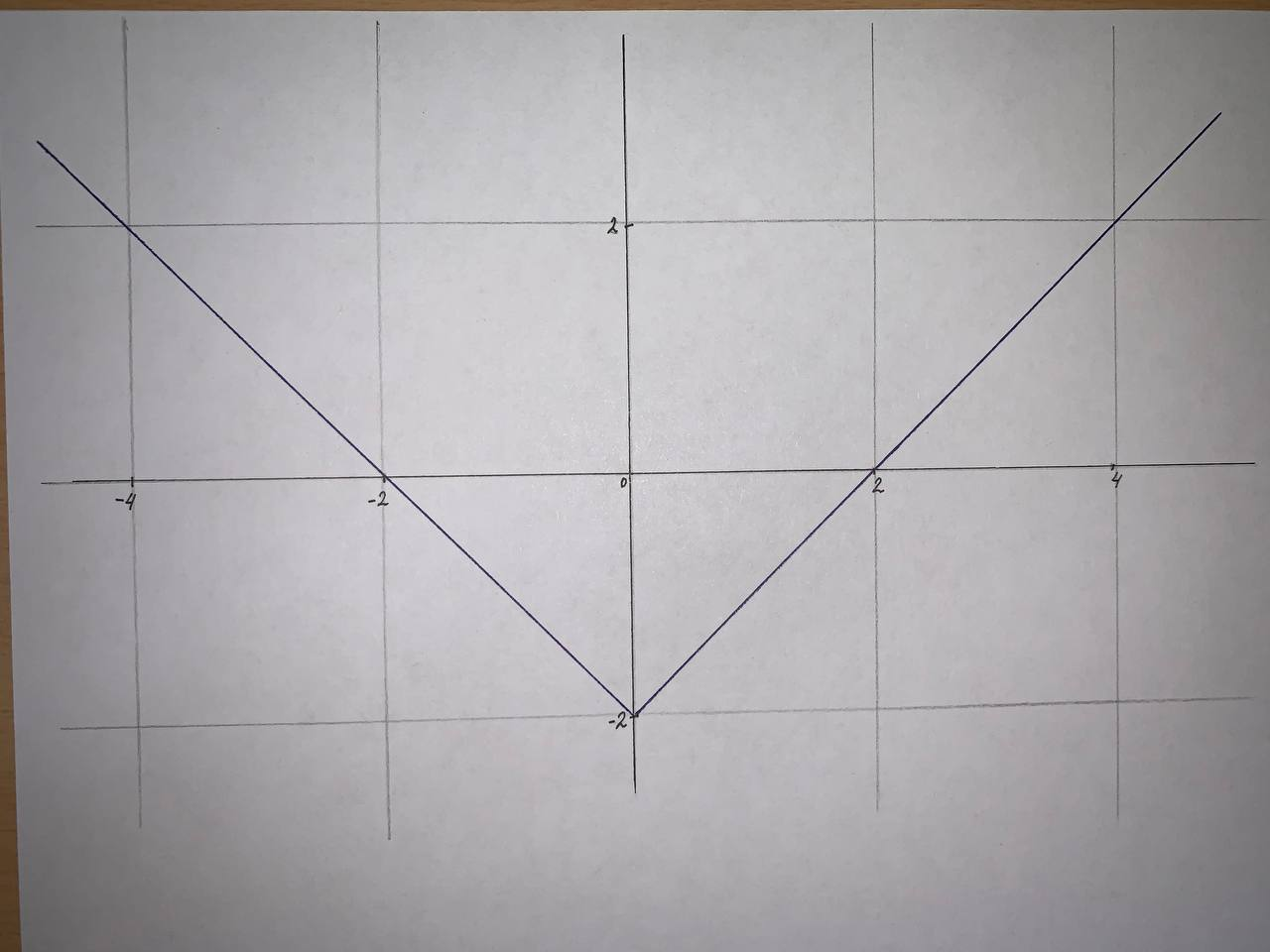Graph the function by hand, not by plotting points, but by starting with the graph of one of the standard functions y=|x|-2necessaryh 2021-09-12 Answered
Graph the function by hand, not by plotting points, but by starting with the graph of one of the standard functions and then applying the appropriate transformations.
$$\displaystyle{y}={\left|{x}\right|}-{2}$$

• Questions are typically answered in as fast as 30 minutes

Solve your problem for the price of one coffee

• Math expert for every subject
• Pay only if we can solve itsmallq9

Step 1
given:
the function $$\displaystyle{y}={\left|{x}\right|}-{2}$$
Step 2
as we know that if we have function y=f(x) then the equation of the function shifted at (h,k) is given by:
$$y-k=f(x-h)$$
the function $$\displaystyle{y}={\left|{x}\right|}-{2}$$ can be rewritten as:
$$\displaystyle{y}={\left|{x}\right|}-{2}$$
$$\displaystyle{y}+{2}={\left|{x}\right|}$$
$$\displaystyle{y}-{\left(-{2}\right)}={\left|{x}\right|}$$
by comparing the equation obtained above with the equation y-k=f(x-h) it can be seen that
h=0
and k=-2
therefore it is the equation of the function $$\displaystyle{y}={\left|{x}\right|}$$ which is shifted at (0,-2).
Step 3
therefore the graph of the function $$\displaystyle{y}={\left|{x}\right|}-{2}$$ can be drawn by shifting the graph of the function $$\displaystyle{y}={\left|{x}\right|}$$ at (0,-2).